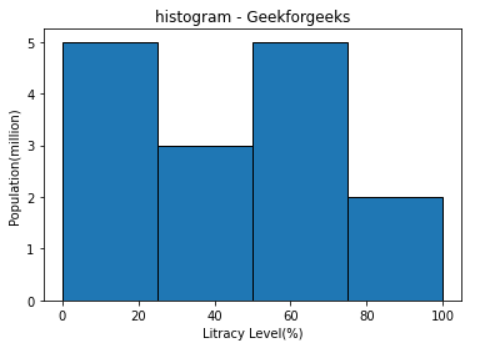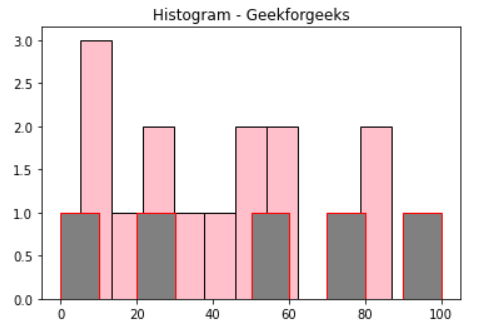Related Articles
Add a border around histogram bars in Matplotlib
• Last Updated : 24 Jan, 2021

Prerequisites: Matplotlib

In this article, we will see how can we can add a border around histogram bars in our graph using matplotlib, Here we will take two different examples to showcase our graph.

Approach:

• Import required module.
• Create data.
• Add border around histogram bars.
• Normally plot the data.
• Display plot.

Below is the Implementation:

Example 1:

In this example, we will Pass an edgecolor = ‘Black’ value as the edge color parameter to plt.hist() to change the bar border color.

## Python3

 `# importing package``from` `matplotlib ``import` `pyplot as plt``import` `numpy as np`` ` `#create data``fig,ax ``=` `plt.subplots(``1``,``1``)``a ``=` `np.array([``22``, ``87``, ``5``, ``43``, ``56``, ``73``, ``55``,``              ``54``, ``11``, ``20``, ``51``, ``5``, ``79``, ``31``, ``27``])`` ` `b ``=` `(``0``, ``25``, ``50``, ``75``, ``100``)`` ` `# Adjust the border color``ax.hist(a, b, edgecolor ``=` `"black"``)`` ` `ax.set_title(``"histogram - Geekforgeeks"``)``ax.set_xlabel(``'Litracy Level(%)'``)``ax.set_ylabel(``'Population(million)'``)`` ` `plt.show()`

Output:Example 2:

In this example, we will Pass a two edge color, edgecolor = ‘Black’ and ‘red’ value as the edge color parameter to plt.hist() to change the bar border color.

## Python3

 `# importing package``from` `matplotlib ``import` `pyplot as plt``import` `numpy as np`` ` `#create data``fig,ax ``=` `plt.subplots(``1``, ``1``)`` ` `a ``=` `np.array([``20``, ``73``, ``55``, ``31``, ``51``, ``5``, ``79``, ``5``,``              ``43``, ``22``, ``87``, ``54``, ``11``, ``56``, ``27``])`` ` `b ``=` `np.array([``0``, ``25``, ``50``, ``75``, ``100``])`` ` `# Adjust the border color``ax.hist(a, edgecolor ``=` `"black"``, color ``=` `'pink'``)``ax.hist(b, edgecolor ``=` `"red"``, color ``=` `'gray'``)`` ` `ax.set_title(``"Histogram - Geekforgeeks"``)``plt.show()`

Output:Attention geek! Strengthen your foundations with the Python Programming Foundation Course and learn the basics.

To begin with, your interview preparations Enhance your Data Structures concepts with the Python DS Course.

My Personal Notes arrow_drop_up